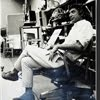## Eugene Ray SDSU 1970'sEugene Ray 1970's. photo credit: Tom Davis

## Wednesday, November 12, 2014

### LEONARDO'S FANTASTIC FORTRESS / blog vision of biotronics

+SDSU BY POPULAR REQUEST /+
>(my aged, worn persona shall<
>focus the blog as work of art)<
+(4PM THURS, ART HALL 512B)+
^^^^^^^^^^^^^^^^^^^^^^
>professor mathew higgins sent a kind<
>message urging my appearance and<
>i shall make the effort thursday to<
+(BLOG FOCUS as (THE+
+NEW BABY) / SLIDES)+
>talk quietly (bronchitis) at sdsu in<
>art lecture hall 512b at 4-6:40 pm<
^^^^^^^^^^^^^^^^^^^^
+SDSU BY DR SUSAN RESNICK+
>(find on the internet /  eugene<
>ray : sdsu library oral history)<
+WOULD BE A GOOD INTRO+
+IT COVERS HISTORY-DATA+
^^^^^^^^^^^^^^^^^^^^

+LEONARDO'S FANTASTIC FORTRESS+
>(circular, compound curve walls<
>were 500 years ahead of others)<
+SUGGESTS HIS COSMIC RESOURCES+
^^^^^^^^^^^^^^^^^^^^^^^^^^^^^
>that has mind boggling value ref<
+(SDSU >GRATITUDE< +
+2014 REUNION BLOG)+
>to leonardo's information source<
>cosmic consciousness his asset<
^^^^^^^^^^^^^^^^^^^^^^
>leonardo da vinci was 500 years early<
>for many of his visionary inventions<
+FROM ET (WHAT ELSE)+
>his fabulous fortress is my favorite< !
^^^^^^^^^^^^^^^^^^^^^^^^
>yes, leonardo did draw this 500 years ahead<
>of schedule and buckminster fuller (friend of<
+/ LEONARDO DA VINCI)+
>isamu noguchi) must have seen it , since it<
>was widely published in the 16th century<
^^^^^^^^^^^^^^^^^^^^^^^^^
>this cathedral design by leonardo in the 16th C<
>shows clearly his value of the circle plan and<
+/ BIOTRONIC ENERGY)+
>conical & spherical roofs which generatate<
>powerful force fields of biotronic energy<
^^^^^^^^^^^^^^^^^^^^^^^^^^^

+LEONARDO'S FORTRESS /+
+FOR CESARE BORGIA 16C+
^^^^^^^^^^^^^^^^^^
>leonardo's low profile, compound curve wall fort<
>has been completely overlooked by architects for<
+/ BORGIA FORTRESS)+
>its amazing technology of an et resourced vision<
>/ there were no other forts anything like this one<
^^^^^^^^^^^^^^^^^^^^^^^^^^^^
>it was the infamous cesera borgia, (brother-in-law<
>to french king francois I who later hired leonardo<
>w/ the aid of count michael de gruyere) who this<
+EXTRATERRESTRIAL VISION)+
>transcendental compound curve brick wall fort<
>was designed for, that defies known technology<
>of a period of time stretching until the 21st cent<
^^^^^^^^^^^^^^^^^^^^^^^^^^^^^^^>remember, the research of wun chok bong, chris<
>dunn and david hatcher childress all agree that<
+>>/ PYRAMID POWER)+
>the ancient pyramids were energy generators<
^^^^^^^^^^^^^^^^^^^^^^^^^^^
>a fairly recent discovery in bosnia, egypt and<
>the yucatan via kerlian photos are the beams<
+/ THE GOD'S MACHINES)+
>of energy emitted by pyramids into the sky<
>corroborating the wun chok bong research<
^^^^^^^^^^^^^^^^^^^^^^^^^^
>my nightly blog via the www internet<
>/>>  eugenerayarchitect.blogspot.com<
^^^^^^^^^^^^^^^^^^^^^^
>is an attempt to synergize life, art, research<
>asking the vital pentagram of questions<….
^^^^^^^^^^^^^^^^^^^^^^
>who are we, where from, where go, what did<
>we do & what did we learn from the process<
^^^^^^^^^^^^^^^^^^^^^^^^^^^
>the art & architecture oeuvre is only part of<
>a long metaphysical history starring davinci<
+METAPHYSIC HISTORY)+
>odilon redon, edgar degas, lecorbusier and<
>many other fascinating persona of history<
^^^^^^^^^^^^^^^^^^^^^^^^^^^^
>russian research engineers & scientists have<
>constructed these prototype study pyramids<
^^^^^^^^^^^^^^^^^^^^^^^^^^^
>"the proof is in the pudding",>for atheists and agnostics<
+DR PATRICK FLANIGAN)+
>costing millions of dollars building & testing pyramids<
>for biotronics which reminds of our work in the 1970s<
^^^^^^^^^^^^^^^^^^^^^^^^^^^^^^^^^
+THIS AFTERNOON I VISIT+
+ART BUILDING WHERE+
+MUCH OF OUR EARLY+
>(sdsu, environ design<
+SDSU ENERGY DESIGN+
+TOOK PLACE, 1969-96+
^^^^^^^^^^^^
>for all my friends there i am<
>very grateful but i must stay<
+(CALM IS PRECIOUS+
+/ I AM GRATEFUL)+ !
>calm/ bronchitis requires it<